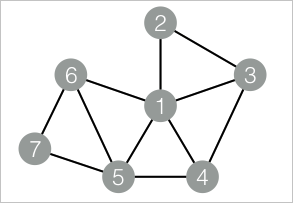A vertex clustering coefficient is used to calculate the peripheral density of a vertex in Undirected Graph G. The density of a star network is 0, and that of a fully meshed network is 1. This topic describes the Vertex Clustering Coefficient component provided by Machine Learning Studio.

You can configure the component by using one of the following methods:

## Machine Learning Platform for AI console

Tab Parameter Description
Fields Setting Start Vertex The start vertex column in the edge table.
End Vertex The end vertex column in the edge table.
Parameters Setting Largest Vertex Degree If the vertex degree is greater than the value of this parameter, sampling is required. This parameter is optional. Default value: 500.
Tuning Workers The number of vertices for parallel job execution. The parallelism level and framework communication costs increase with the value of this parameter.
Memory Size per Worker (MB) The maximum size of memory that a single job can use. By default, the system allocates 4,096 MB for each job. If the used memory size exceeds the value of this parameter, the OutOfMemory exception is reported.
Data Split Size (MB) The data split size. Default value: 64.

## PAI command

``````PAI -name NodeDensity
-project algo_public
-DinputEdgeTableName=NodeDensity_func_test_edge
-DfromVertexCol=flow_out_id
-DtoVertexCol=flow_in_id
-DoutputTableName=NodeDensity_func_test_result
-DmaxEdgeCnt=500;``````
Parameter Required Description Default value
inputEdgeTableName Yes The name of the input edge table. No default value
inputEdgeTablePartitions No The partitions in the input edge table. Full table
fromVertexCol Yes The start vertex column in the input edge table. No default value
toVertexCol Yes The end vertex column in the input edge table. No default value
outputTableName Yes The name of the output table. No default value
outputTablePartitions No The partitions in the output table. No default value
lifecycle No The lifecycle of the output table. No default value
maxEdgeCnt No If the vertex degree is greater than the value of this parameter, sampling is required. 500
workerNum No The number of vertices for parallel job execution. The parallelism level and framework communication costs increase with the value of this parameter. Not configured
workerMem No The maximum size of memory that a single job can use. By default, the system allocates 4,096 MB for each job. If the used memory size exceeds the value of this parameter, the OutOfMemory exception is reported. 4096
splitSize No The data split size. 64

## Examples

1. Generate training data.
``````drop table if exists NodeDensity_func_test_edge;
create table NodeDensity_func_test_edge as
select * from
(
select '1' as flow_out_id, '2' as flow_in_id from dual
union all
select '1' as flow_out_id, '3' as flow_in_id from dual
union all
select '1' as flow_out_id, '4' as flow_in_id from dual
union all
select '1' as flow_out_id, '5' as flow_in_id from dual
union all
select '1' as flow_out_id, '6' as flow_in_id from dual
union all
select '2' as flow_out_id, '3' as flow_in_id from dual
union all
select '3' as flow_out_id, '4' as flow_in_id from dual
union all
select '4' as flow_out_id, '5' as flow_in_id from dual
union all
select '5' as flow_out_id, '6' as flow_in_id from dual
union all
select '5' as flow_out_id, '7' as flow_in_id from dual
union all
select '6' as flow_out_id, '7' as flow_in_id from dual
)tmp;
drop table if exists NodeDensity_func_test_result;
create table NodeDensity_func_test_result
(
node string,
node_cnt bigint,
edge_cnt bigint,
density double,
log_density double
);``````
The following figure shows the structure of the vertex clustering coefficient graph.2. View training results.
``````1,5,4,0.4,1.45657
2,2,1,1.0,1.24696
3,3,2,0.66667,1.35204
4,3,2,0.66667,1.35204
5,4,3,0.5,1.41189
6,3,2,0.66667,1.35204
7,2,1,1.0,1.24696``````